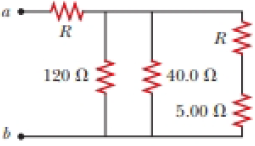# The resistance between terminals a and b in Figure P27.36 is 75.0 Ω. If the resistors labeled R have the same value, determine R . Figure P27.36### Physics for Scientists and Enginee...

9th Edition
Raymond A. Serway + 1 other
Publisher: Cengage Learning
ISBN: 9781305116399

#### Solutions

Chapter
Section### Physics for Scientists and Enginee...

9th Edition
Raymond A. Serway + 1 other
Publisher: Cengage Learning
ISBN: 9781305116399
Chapter 28, Problem 28.56AP
Textbook Problem
221 views

## The resistance between terminals a and b in Figure P27.36 is 75.0 Ω. If the resistors labeled R have the same value, determine R.Figure P27.36To determine
The value of resistance R .

### Explanation of Solution

Given Information: The resistance between terminals points a and b is 75.0Ω .

The resistors R and R5.00Ω are connected in series combination.

Formula to calculate the resistance across the circuit when resistors R and R5.00Ω are connected in series combination.

RS=R+R5.00Ω (1)

Here,

RS is the resistance across the circuit when resistors R and R5.00Ω are connected in series combination.

R is the value of resistance R .

R5.00Ω is the value of resistance 5.00Ω .

Substitute 5.00Ω for R5.00Ω in equation (1) to find RS ,

RS=R+5.00Ω

Thus, the resistance across the circuit when resistors R and R5.00Ω are connected in series combination is R+5.00Ω .

The resistors R40.0Ω , R120Ω and RS are connected in parallel combination as shown in figure.

Figure (1)

Formula to calculate the resistance when the resistors are connected in parallel.

1RP=1R40.0Ω+1R120Ω+1RS (2)

Here,

RP is the value of the resistance when the resistors are connected in parallel.

R120Ω is the value of resistance 120Ω .

R40.0Ω is the value of resistance 40.0Ω .

Substitute 120Ω for R120Ω , 40.0Ω for R40.0Ω , R+5.00Ω for RS in equation (2) to find RP ,

1RP=140.0Ω+1120Ω+1R+5

### Still sussing out bartleby?

Check out a sample textbook solution.

See a sample solution

#### The Solution to Your Study Problems

Bartleby provides explanations to thousands of textbook problems written by our experts, many with advanced degrees!

Get Started